Microsoft Excel quick and easy

New Excel tutorial every week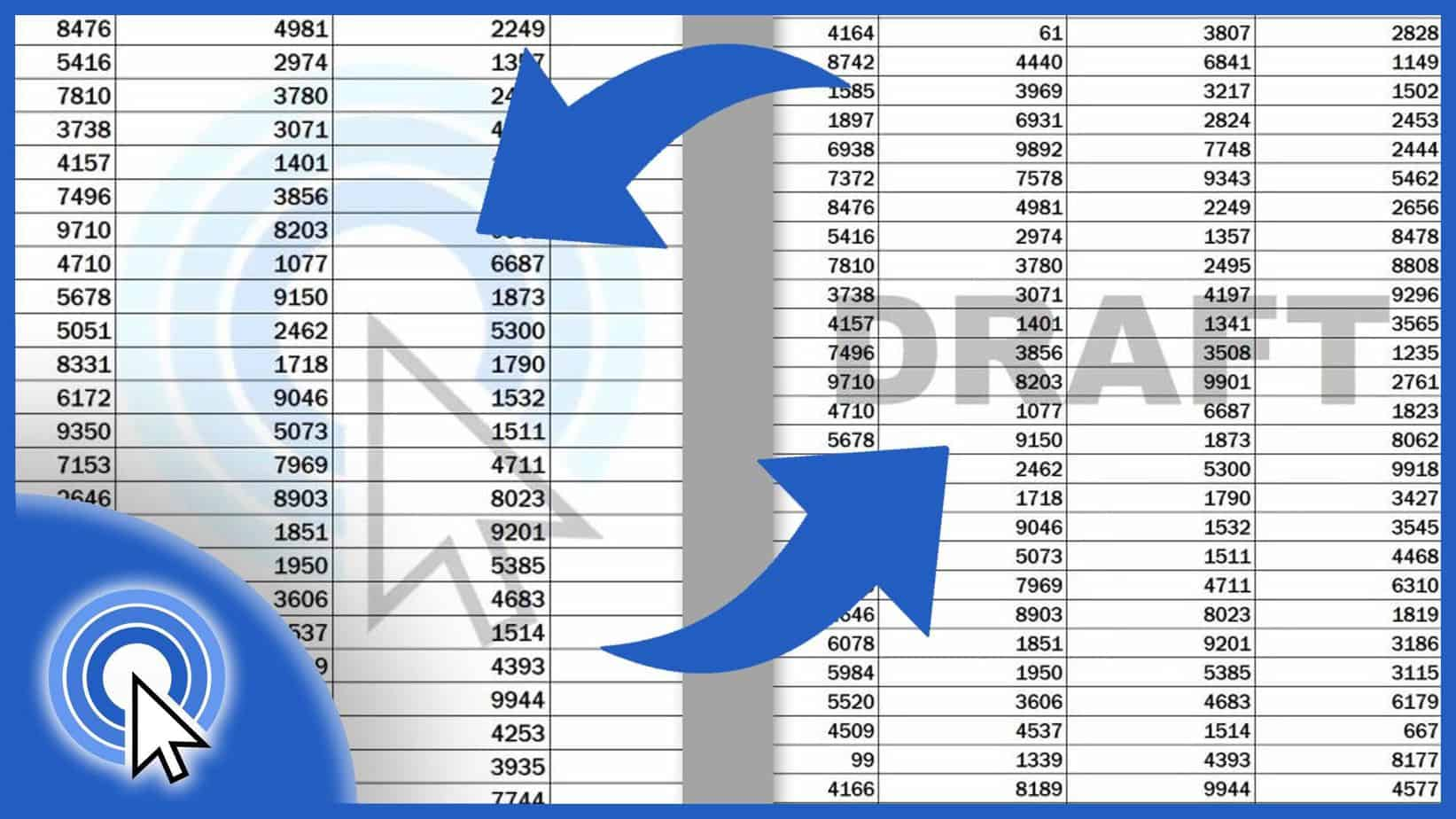## How to Insert a Watermark in Excel (Picture or Text)

Today’s tutorial will be on how to insert a watermark in Excel. We’re going to go through a quick and easy way to do that.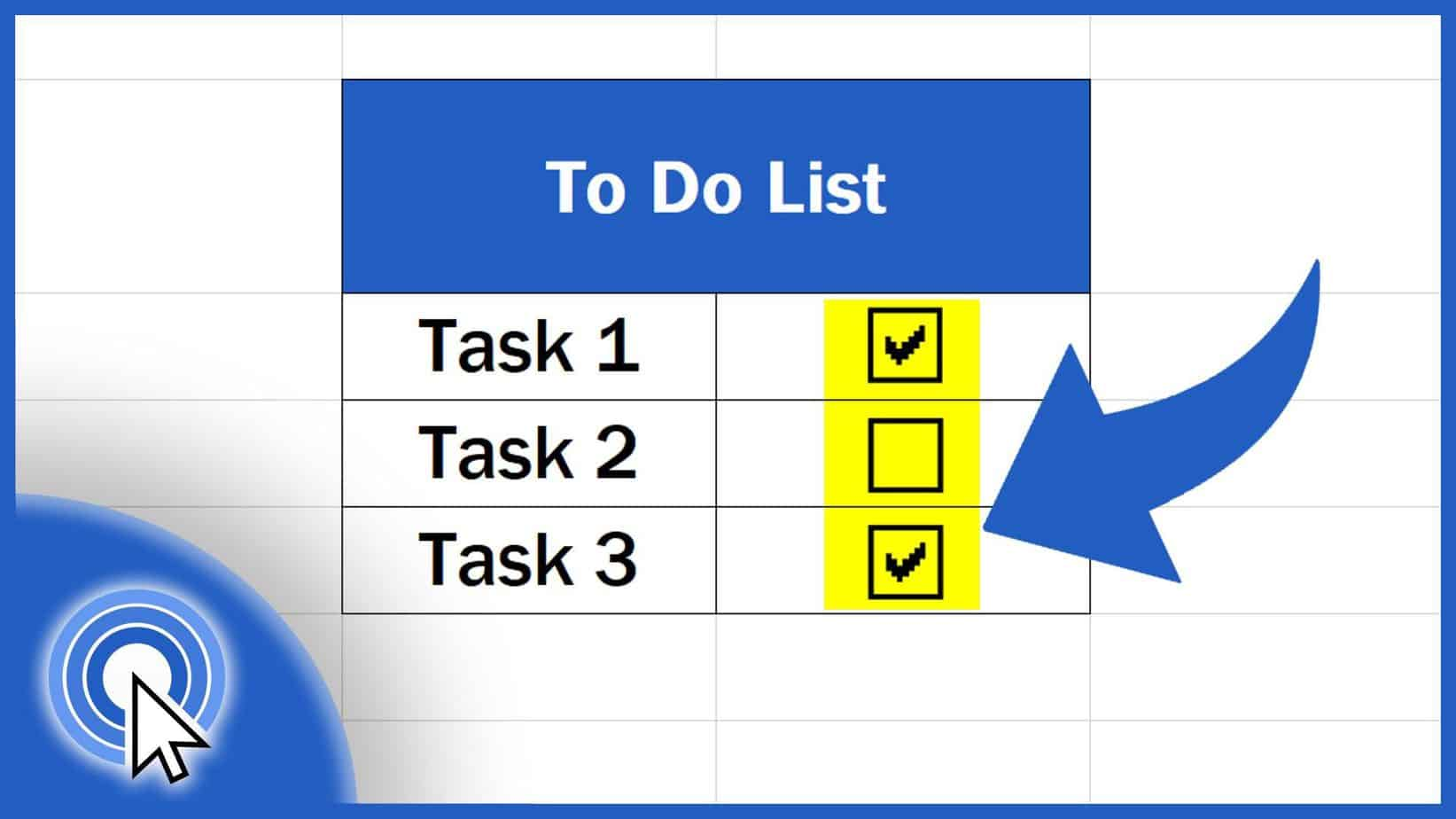## How to Insert a Checkbox in Excel (Quick and Easy)

In this tutorial, we’re going to talk about how to insert a checkbox in Excel in a quick and easy way.## How to Calculate a Rank in Excel (Descending / Ascending)

In this tutorial, we’re going to have a look at how to calculate a rank in Excel and sort the numbers in ascending or descending order.## How to Calculate the Median in Excel (Quick and Easy)

Today we’re going to go through how to calculate the median in Excel. You’ll see a easy way to find out the midpoint in any set of numbers.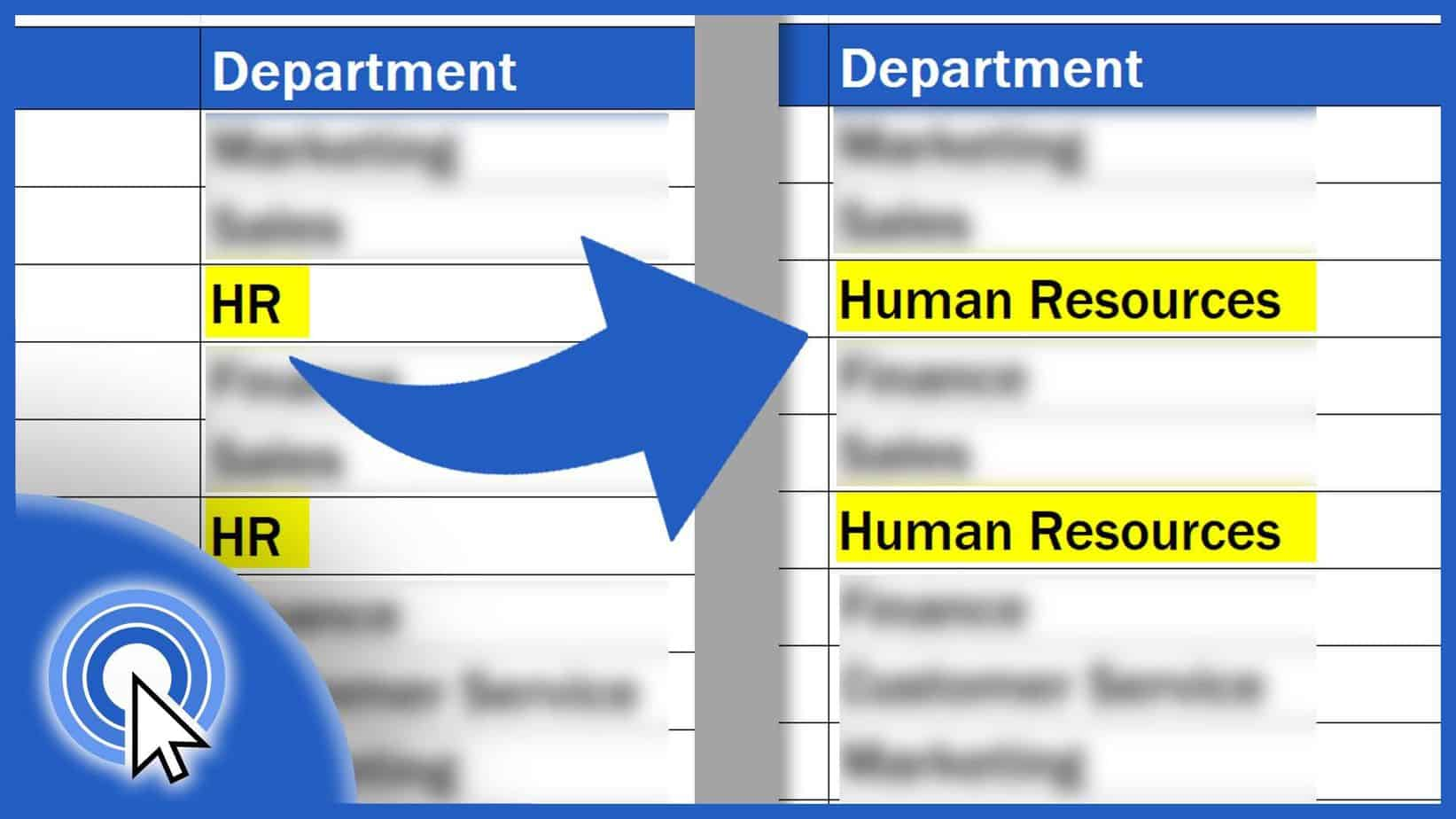## How to Replace Words in Excel (and avoid unnecessary mistakes)

Would you like to know how to replace words in Excel in a convenient way and avoid unnecessary mistakes? Then you’re in the right place.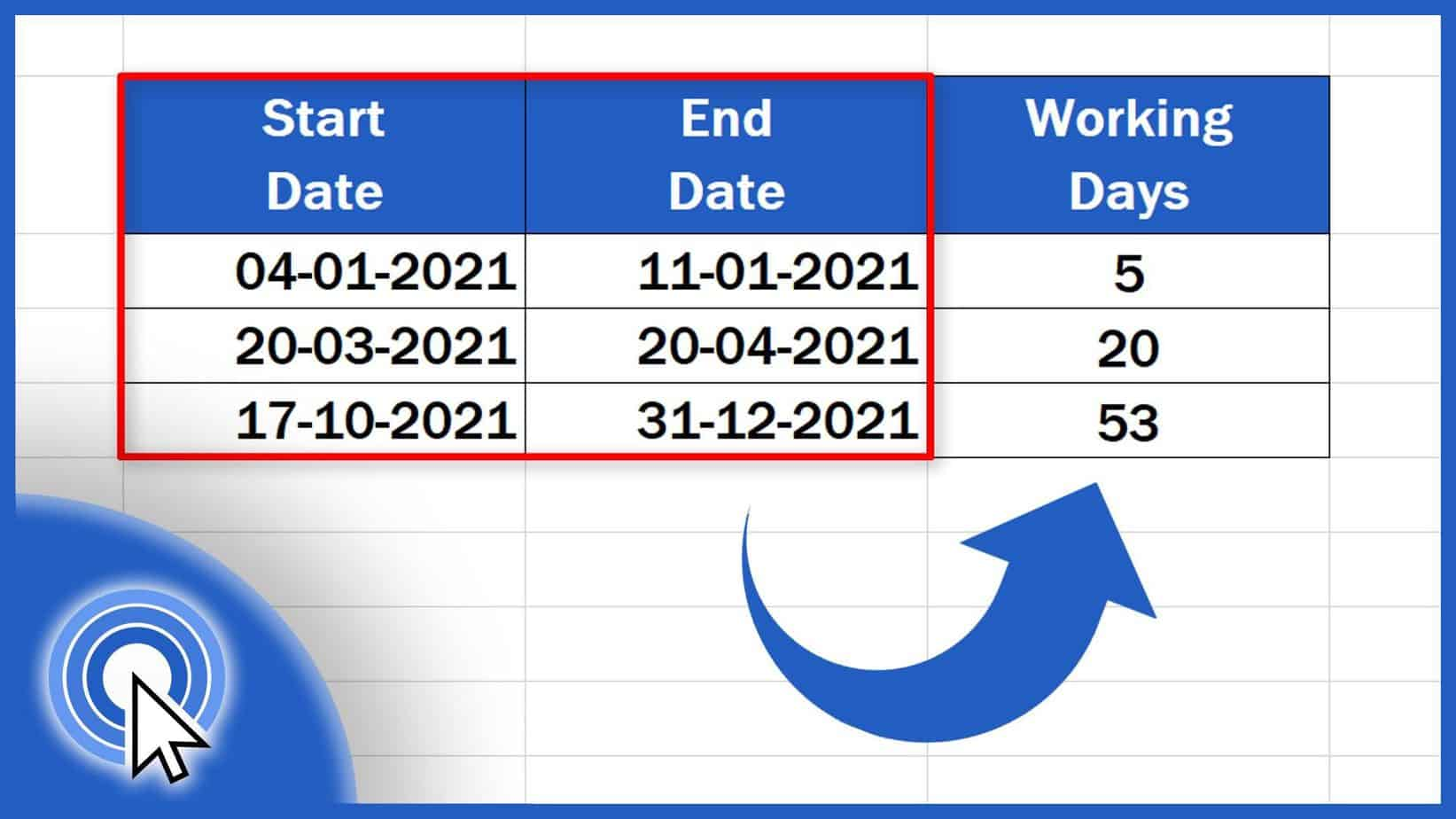## How to Calculate Working Days in Excel (Two Helpful Ways)

Today’s video tutorial describes two super guick, easy and helpful ways how to calculate working days in Excel.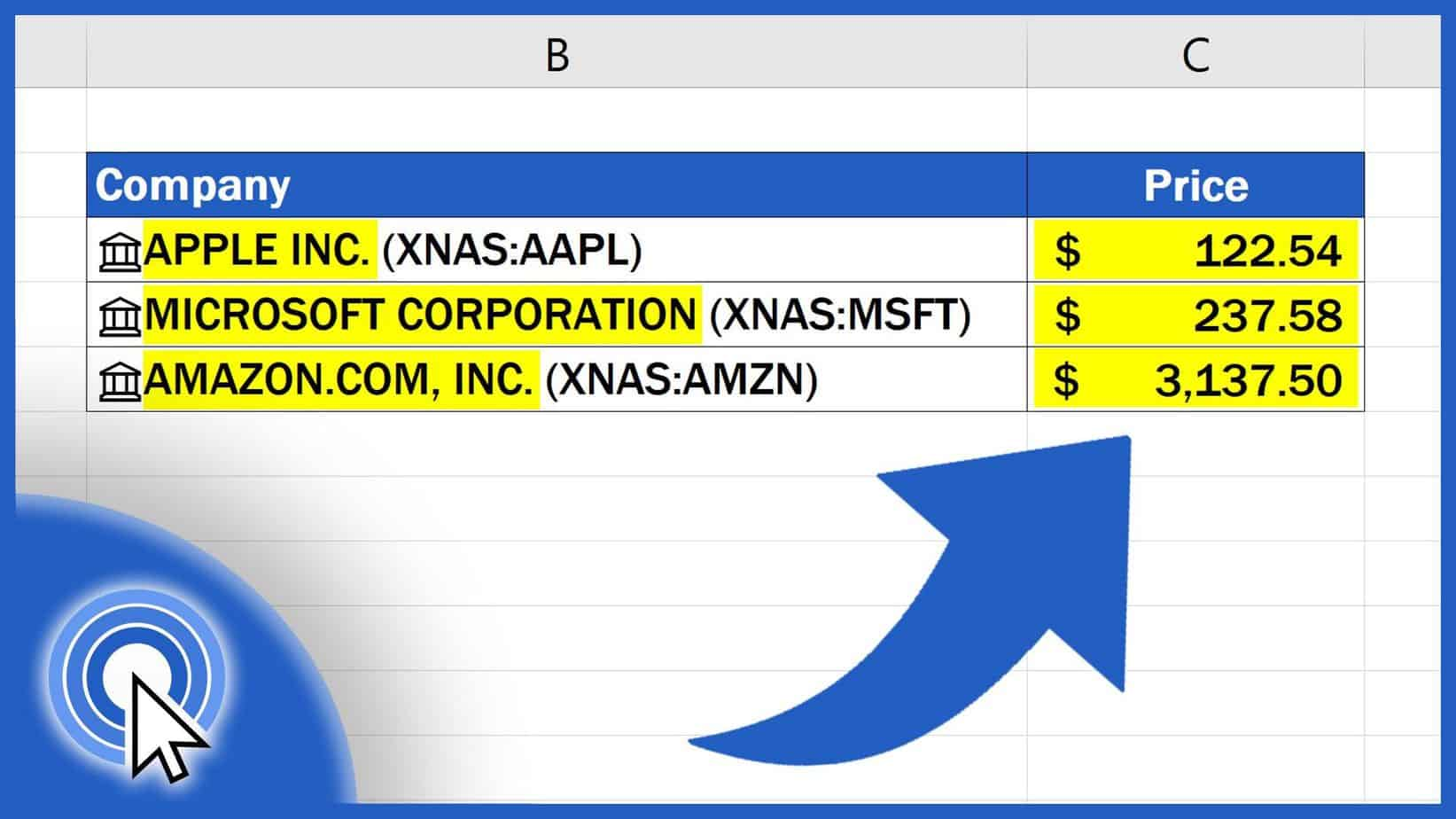## How to Get Stock Prices in Excel (Free and Automated Solution)

In this simple tutorial, we’re going to have a look at how to get stock prices in Excel easily and for free.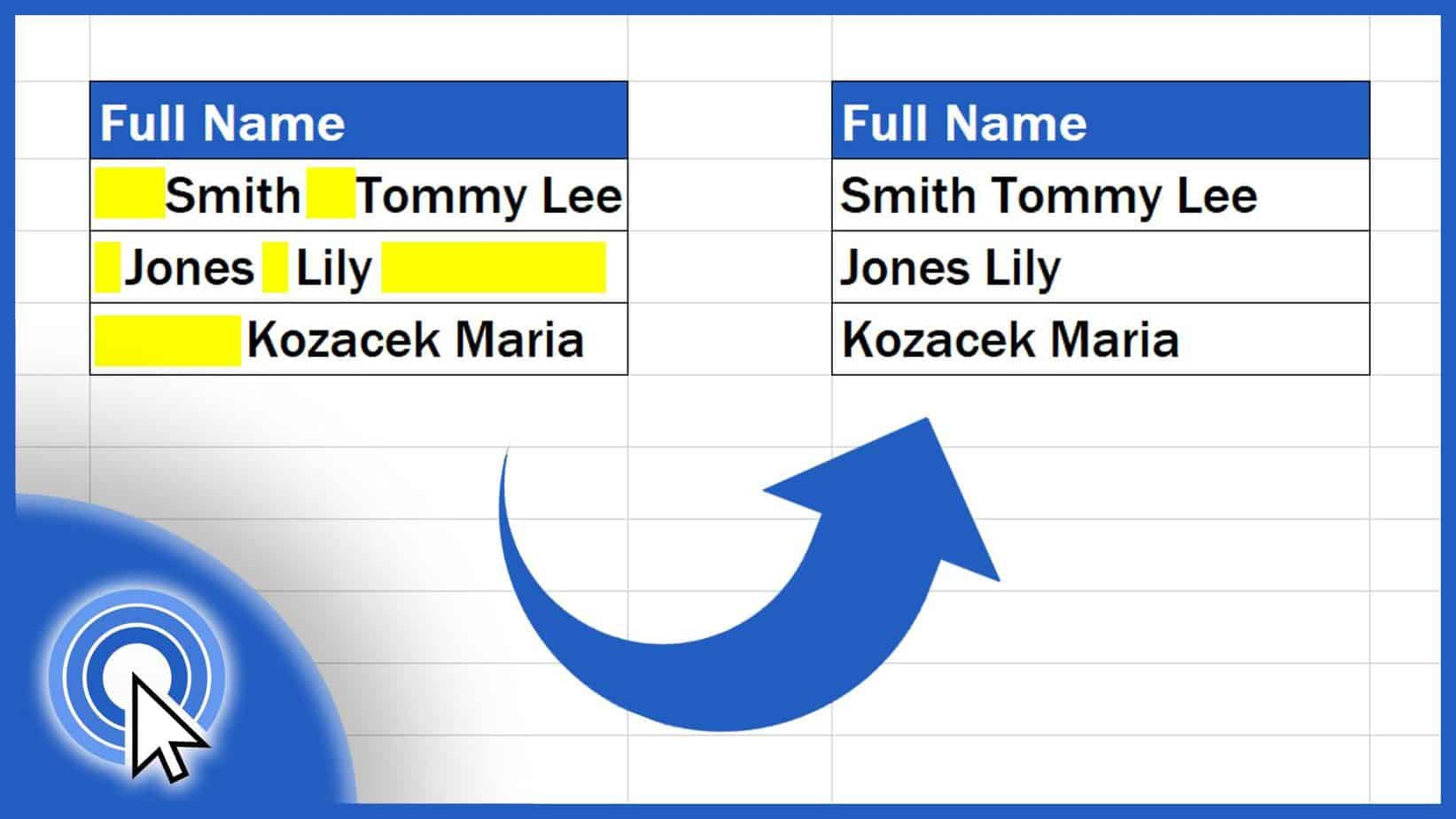## How to Remove Spaces in Excel (Two Useful Ways)

In today’s tutorial, we’re gonna go through two helpful and easy ways how to remove blank spaces in Excel.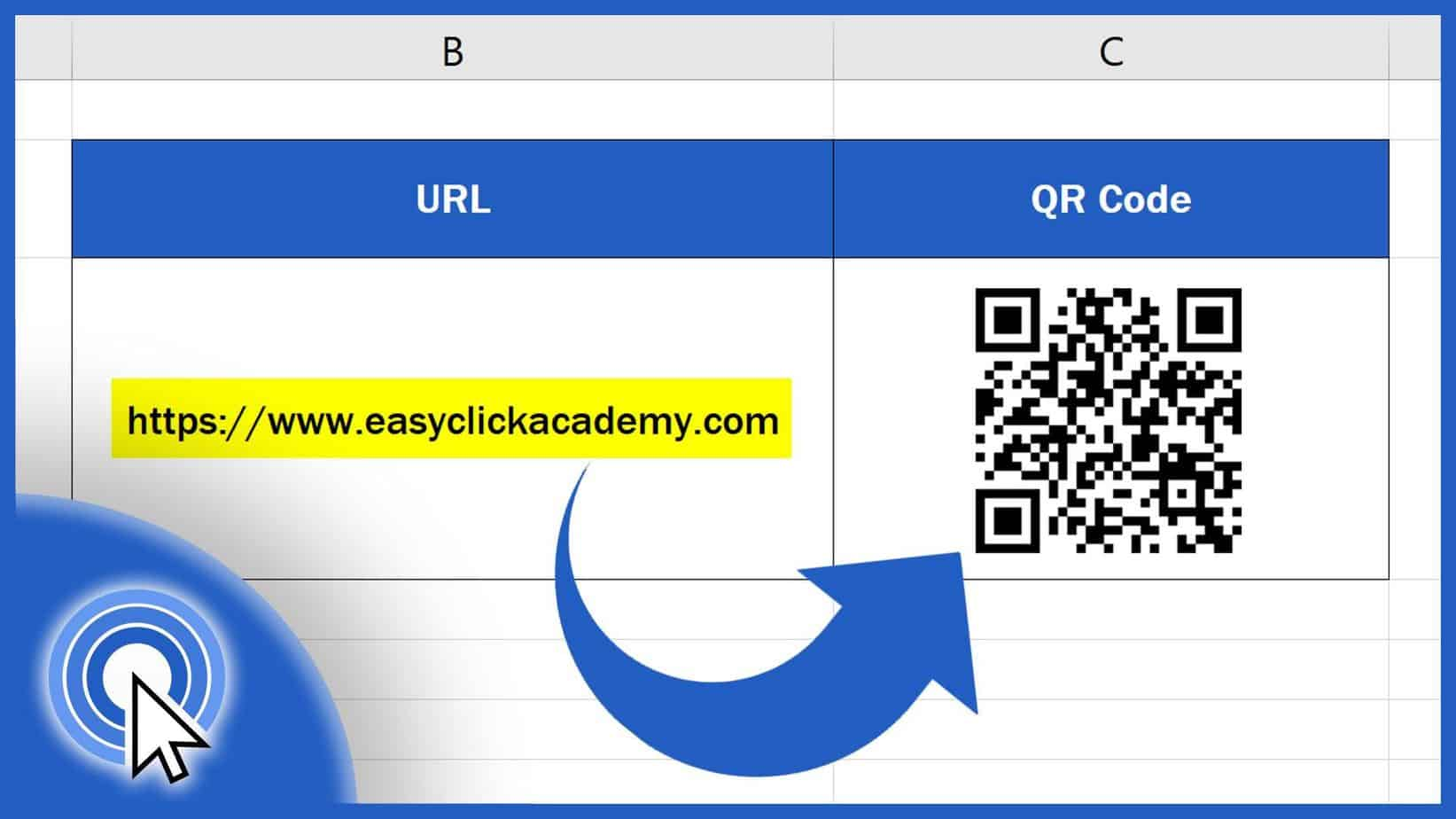## How to Create a QR Code in Excel (Free and Simple)

In this quick tutorial, we’ll be having a look at how to create a QR code in Excel in a free and simple way.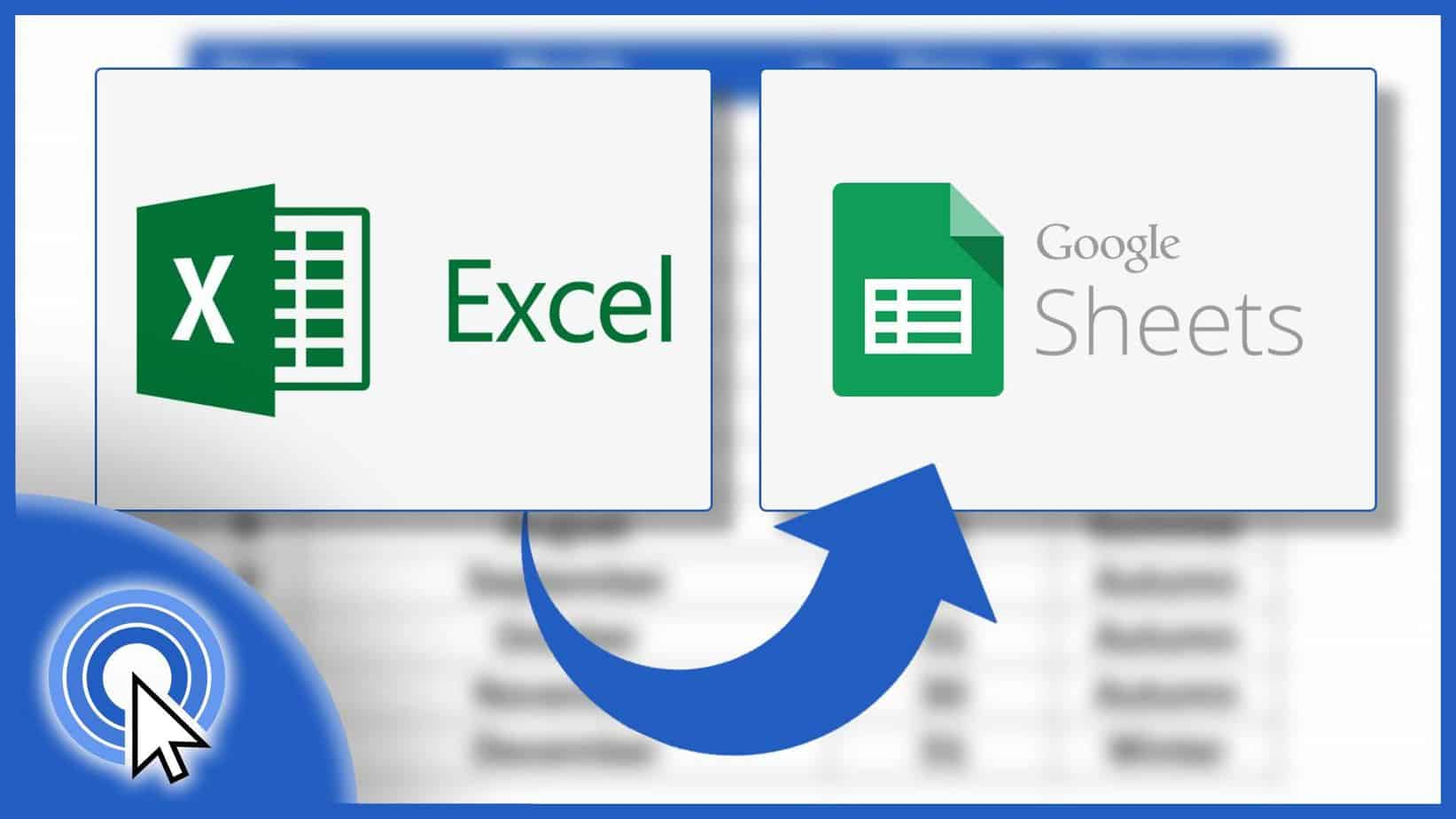## How to Convert Excel to Google Sheets (Quick and Easy)

In this tutorial, we’re gonna go through how to convert Excel file to Google Sheets document in a quick and easy way.